# Net Present Value (NPV): What is it?Reviewed by

Patrick Curtis WSO Editorial Board

Expertise: Investment Banking | Private Equity

Net Present Value (NPV) is a financial term used to define the value of the total of all cash flows (both present and future) discounted to the present and is frequently used as part of capital budgeting.

Organizations usually require large capital investments as part of their plan to expand operations and seek new projects to undertake. They must make decisions regarding investments in such projects very carefully, undertaking the most profitable projects. Therefore, capital budgeting tools, such as Net Present Value (NPV), are utilized to understand if a project will turn out to be profitable or not, and also to compare the profitability between different projects.Money received or paid in the future is usually worth less than in the present, and so a discount rate is applied (usually a risk-free interest rate) to determine what the present value is. This is due to a popular concept called the time value of money (TVM).

The following video provides a great explanation of the concept of TVM.

More specifically, NPV is the difference between the present value of future cash inflows and outflows and is used to determine the profitability and feasibility of an investment in a project. If the NPV is positive, the project is worth undertaking and vice versa.

A positive NPV means that the present value of future cash inflows, or the future revenue, is greater than the present value of cash outflows, or the costs. The opposite is true for a negative NPV. An NPV of zero means the present value of future cash inflows is equal to the present value of cash outflows at the rate of interest used to discount them. This rate of interest which equates the NPV to zero is known as the internal rate of return (IRR). The Net Present Value Rule dictates that only projects with a positive NPV should be considered, as they are the projects that will generate a positive return for the business.## Why discount cash flows to calculate NPV?

Money in the future is usually worth less than money today. This is the basis for the concept of the TVM. Future cash flows are discounted to present value as:

1. Money in the future has reduced buying power due to the effects of inflation, which decreases the purchasing power of money due to the increase in prices of goods and services
2. Money today can be invested to earn a specific rate of return, which is generally greater than or equal to the risk-free rate of return in the economyFurthermore, cash flows are also discounted to account for the relative risks of a certain project. All projects carry different risks, and to account for this risk, discount rates vary depending on the relative risk, with high-risk projects carrying higher discount rates.

Therefore, cash flows must be discounted to arrive at the Net Present Value of an investment taking into account the relative risk of a project, the time value of money, the opportunity costs, as well as the rate of inflation.## Limitations of the NPV Approach

Although the NPV approach has its many advantages, such as accounting for the time value of money, it also has its fair share of shortfalls that hinder the effectiveness and reliability of the approach. Some of these limitations include the difficulty in calculating the required rate of return due to unclear guidelines, and the inability to use net present value when comparing projects or investments of different sizes. It is important that businesses consider these limitations, and use net present value along with other capital budgeting tools, such as the internal rate of return, to truly understand the economic feasibility of capital investment, and whether it is worthwhile for the business to undertake.

### Calculating the required rate of return

The required rate of return, or the cost of capital, is the minimum return investors require for the risk undertaken by them for that project or investment. Despite being the most important measure, there are no set guidelines on how to calculate this value. Because of this, some businesses may use a cost of capital that is too high, which would lead to them missing out on a potential opportunity, or may use a cost of capital that is too low, which would then make an investment that is not worthwhile look good.

The most widely used rate to discount cash flows is the weighted average cost of capital (WACC). As this is the cost that the business pays on its sources of capital, it would be unacceptable to accept any investment opportunity below this rate. If the NPV calculated using this rate is negative, the business must not invest as it means that the business is destroying value to its stakeholders.

### Comparing projects/investments of different sizes

As the NPV is an absolute dollar figure, the NPV of projects with different sizes will not be comparable. Projects that are larger, but have lower rates of returns, will have a higher net present value than projects that are smaller in size but provide a better rate of return.

For example, Project A requires the business to invest \$700,000, and has an NPV of \$300,000, while Project B requires the business to invest \$100,000, and has a net present value of \$90,000. Even though the NPV of Project A is higher, project B has a higher return on investment. So when reviewing the net present value of these 2 projects, Project A looks like the better project, despite project B having a higher rate of return on investment.

This is one of the major limitations of the NPV approach and greatly hinders its ability to provide accurate representations for businesses of different sizes.

The video below provides a brief explanation of some of the advantages and disadvantages of the NPV approach:

## NPV vs IRR

The internal rate of return (IRR) method is another capital budgeting tool used to evaluate the profitability of projects where, instead of assigning a discount rate such as the NPV method, it calculates the discount rate required in order to break even. Essentially, it will calculate the discount rate the project must have in order for the present value of all cash flows of the project to equate to the initial investment in the project (i.e. where NPV = 0).

Once the IRR is calculated, it must be compared to the actual discount rate of the project. If the IRR is above the discount rate of the project, then the project is worthwhile to undertake. If the IRR is below the discount rate, then the project is considered to not be financially worthwhile. The IRR method is most commonly used by Private Equity firms and Hedge Funds to evaluate potential opportunities.

Let us understand this with an example. Autodeck Pty Ltd wants to undertake a particular project and needs help in determining whether it is worthwhile to undertake. Autodeck has determined that the project will require a \$130 million capital outlay, but, when discounted at a 20% annual rate, generates annual cash flows of \$50 million. Autodeck has also mentioned that the project will only last 5 years.

We can illustrate this information with a tableIn order to determine whether the project is worthwhile or not, the IRR must be calculated and compared against the discount rate of 20%. Using the IRR function in excel, we can calculate the value of IRR. This is done by:

• typing =IRR on a cell
• Pressing tab
• Dragging all the cash flow values (including at time 0)
• Pressing enter

This is what your equation should look like in Excel (although it may differ depending on the cells you use):
=IRR(C3:H3)

This results in an IRR of 26.7%. As the required rate of return for the project is 20%, the IRR method tells us that the project is worthwhile to undertake.

### Advantages of the IRR method

1. The discount rate is not necessary for the calculation

Unlike the NPV method, a discount rate is not required to calculate the IRR. For the NPV method, a discount rate is necessary for the calculation of the NPV, and this is usually very difficult to calculate accurately. However, the IRR value will remain the same, no matter what the discount rate is, significantly reducing the risk of using an inaccurate rate. Despite this, a rough idea of the rate of required return will still be required in order to compare it to the IRR and evaluate if the project is feasible or not.

2. Is simplistic

The IRR is fairly simple to interpret. Managers like using the IRR to evaluate projects for investment because it is extremely easy to interpret once calculated - if the IRR exceeds the required rate of return, accept the project, otherwise, reject it.

### Disadvantages of the IRR method

1. Inaccurate for projects of different sizes

One of the largest pitfalls of the IRR method is its inability to accurately rank projects based on their IRR when the projects are of different sizes. The IRR method will favor a small project with a larger return on investment than a much larger project with a more conservative return on investment.

For example, imagine project A has an NPV of \$3,000,000 and an IRR of 10%, while project B has an NPV of \$200,000 and an IRR of 40%. Even though Project A has the larger dollar benefit of \$300,000, as compared to project B with only an \$80,000 benefit, the IRR method will still rank project B first, simply because the IRR of 40% in project B is higher than the IRR of 10% in project A. Hence, it may not be suitable to compare vastly different sizes of investment opportunities.

2. Reinvestments are not accounted for

The IRR method assumes that future cash inflows can be invested at the same rate as the IRR. This is quite impractical when a project's IRR is large, as opportunities to make investments that would generate the same rates of returns can be quite rare. This assumption may provide an inaccurate representation of project returns.

## NPV vs Payback Period

The payback period is a capital budgeting tool used to evaluate the attractiveness of a potential business project. The main difference between the NPV method and the payback method is that NPV evaluates the value of a project in terms of its' financial returns, whereas the payback method evaluates the value of a project by calculating the period, in years, required for the project to pay back the cost of the initial investment.

An investor who uses the payback method would choose a specific period for the project to break even and return the initial investment. For example, let's look at an investor who is using a payback period of 5 years as the criterion for accepting projects. If a certain project's payback period was longer than 5 years, the company would reject it, and if it was shorter than 5 years, the company would accept it. The shorter the payback period, the more worthwhile it is to pursue the project.

The formula to calculate the payback period is as follows:Let us look at another example to understand how we could calculate the payback period.

Autodeck Pty Ltd wants to determine whether or not it should undertake a new project regarding energy conservation. It has been informed that the Capital Expenditure costs to acquire the project would be \$2,300,000 and is expected to yield annual cash flows of \$328,500.

To calculate the payback period, we simply divide the Capex by annual cash flows.Assuming the criterion for accepting projects is 5 years, Autodeck will reject this project as its returns will not cover the initial investment within 5 years.

1. Accounts for tax and the depreciation tax shield

The payback method is advantageous as it factors in tax and depreciation tax shield. The annual cash flows are measured as after-tax cash flows, and the effect of depreciation tax shield (which reduces tax liability as depreciation is generally a tax-deductible expense), is also included.

2. Simple to calculate

Another advantage of the payback method is that it is a much simpler alternative to the NPV method. All you need to calculate the payback period is the Capex costs and the expected annual cash flows.

1. Ignores inflation and the time value of money

The main disadvantage of the payback method vs the NPV method is that it does not take into account the time value of money, and ignores the impact of inflation. Under the payback method, \$1 today is equal to \$1 sometime in the future, which is simply not true as inflation reduces the purchasing power of money. So \$1 today will be worthless in the future due to the increase in the prices of goods and services.

2. Ignores future cash flows beyond the project's payback period

Additionally, the payback method ignores the cash flows that are beyond the payback period of the company. Typically, the projects that have the most significant returns often require that a lot of time is invested into it. The payback method doesn't take these cash flows into account and only accounts for the cash flows that occur within the payback period.

As both the payback method and the NPV method have their advantages and disadvantages, businesses often combine these capital budgeting tools when analyzing the return on investment (ROI) of the various opportunities under consideration.

## Calculating Net Present Value

There are 2 formulas that can be used to calculate the net present value of a project, which are: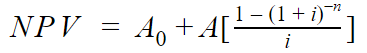Where:

• A0 is the initial cost of investment
• A is the annual cash flow
• i is the discount rate or the required rate of return
• t is the number of periods there are for the project

What is interesting in this formula is the term inside the square brackets is the formula for the present value of an annuity. The second formula that can be used to calculate NPV is: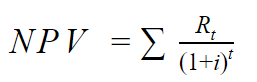Where:

• Rt is the net cash flow at time t
• i is the discount rate or the required rate of return
• t is the number of periods there are for the project

## Example of NPV

A mining project requires a \$2m upfront investment and gains \$4.5m every year in revenue. Its incremental costs are \$1.5m every year, and it has a required rate of return of 10%. You are offered an opportunity to take on this project for 10 years. How do you calculate and decide if it is worthwhile?

The \$2m upfront investment is the Capital Expenditure (Capex) required at time 0, with incremental revenues of \$4.5m every year, and incremental costs of \$1.5m every year, for 10 years. We can display this information as a table: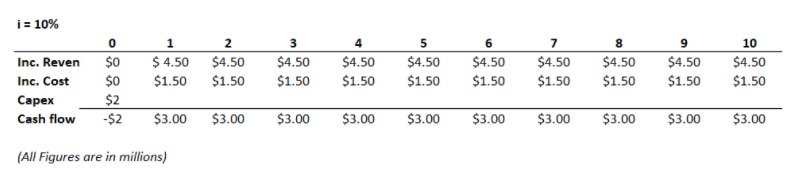After realizing the incremental revenues and cost, and Capex, we can demonstrate the cash flow, which is at negative \$2 million for time 0, and then is at \$3 million consistently for 10 years (calculated as incremental revenue - incremental cost).

Now, we can use the first formula introduced:Where,

• A0 = -2
• A = 3
• i = 10%
• n = 10 years

Therefore, we get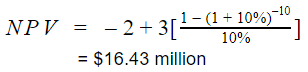The net present value of this project, calculated by discounting the cash flows back by 10 years, is \$16.43 million, hence, the project is worthwhile to undertake as it provides a good return for the business

But what if the cash flows in the future are not constant? In that case, you have to manually discount each cash flow back to the present value and sum it up to arrive at the NPV. The following table illustrates how this is done: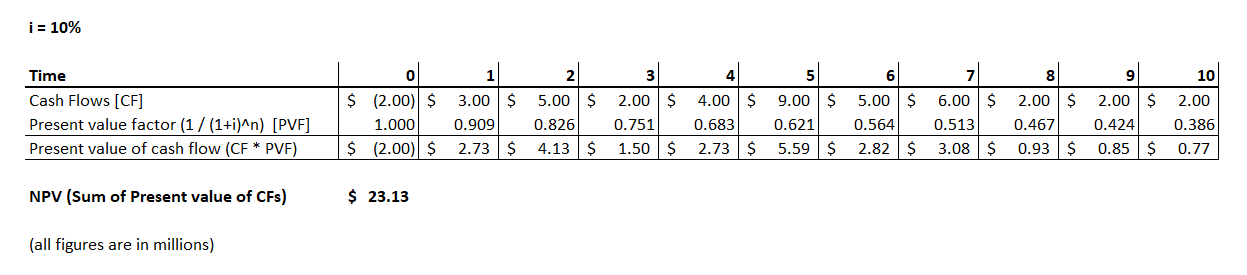The following video provides another great example that will help consolidate the information we have learned:Module 1: Introduction
Module 2: DCF - The Big Picture
Module 3: Enterprise Value & Equity Value Practice
Module 4: DCF Introduction
Module 5: DCF Fundamentals
Module 6: WACC Overview
Module 7: Deriving Share Price From the NPV of Future Cash Flows
Module 8: Nike DCF ModelingModule 1: Getting Started
Module 2: Fundamental Concepts
Module 3: The Income Statement
Module 4: Working Capital
Module 5: PP&E and Intangibles
Module 6: The Cash Flow Statement
Module 7: Debt & Interest Schedule
Module 9: BonusModule 1: Introduction
Module 2: Valuation: The Big Picture
Module 3: Enterprise Value & Equity Value Practice
Module 5: Trading Comps: The Setup
Module 10: Trading Comps: Benchmarking and Outputs
Module 11: Precedent Transactions: Introduction
Module 12: Precedents: The Setup
Module 13: Spreading Tiffany & LVMH